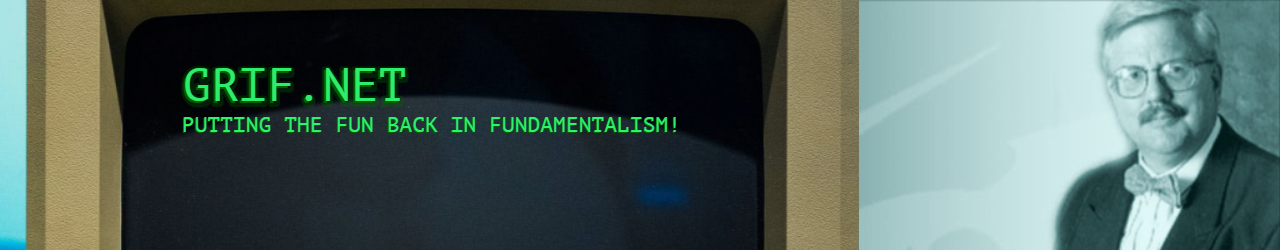# 07/19/21 Grif.Net – Monday Quiz: English Words

## 07/19/21 Grif.Net – Monday Quiz: English Words

English is a =
polyglot language.  1000 years ago there were less than 50,000 =
words used in England (roughly divided into Anglo-Saxon root, Germanic =
root and French/Latin root).  Now there are more than a million =
words.  200,000 are used “commonly” and of these =
“commonly used” words, 43 comprise HALF!  More =
astounding, of those 43 words, just 9 account for 25% of the words =
English speakers use!!

1. List the 9 =
most commonly-used words in English. (any order)

a =

b =

c =

d =

e =

f =

g =

h =

i

2. Almost half =
of all words commonly used are verbs. Try your hand at listing the 12 =
most common verbs (any order)

a =

b =

c =

d =

e =

f =

g =

h =

i

j =

k =

l =

Now some =
easier questions:

3. How many =
distinct sounds are in English?

a Vowels =
__________

b Consonants =
_________

4. What is the =
most common vowel sound? ____________

5. What letter =
combination has more pronunciations than any other?  =
_____________

6. The single =
syllable that sounds like “air” can be spelled in how many =
ways? _________

7. What =
percentage of English words are NOT spelled phonetically (the way they =
sound)? ____%

8. What =
5-letter word is the only word that retrains its pronunciation even if 4 =
letters are removed?

9. What three =
English words end in “-gry”?

a

b

c

10. Is there =
any English word that uses all the vowels including “y”? =
____________________

~~

Dr Bob Griffin =

bob@grif.net =
www.grif.net

"Jesus =
Knows Me, This I Love!"